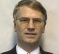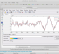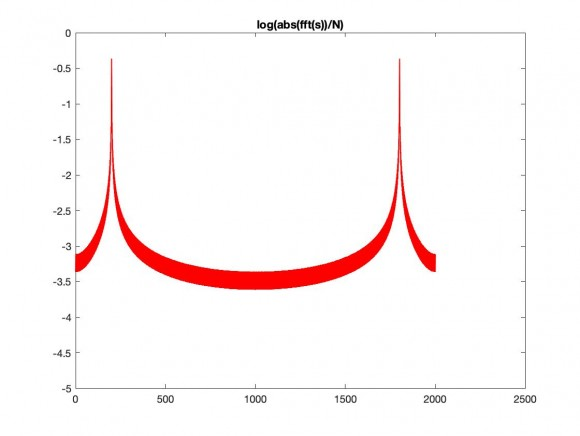## Phase brake in tonal signal and frequency estimationStarted by 2 years ago13 replieslatest reply 2 years ago168 views

Hello! I noticed that breaking phase in tonal signal causes frequnecy component "motion" in spectrum. Could you explain this effect and also interesting how to compensate it. Here' s an example. Thanks!clear

Fs = 48e3;
dt = 1/Fs;
NSIG = 2^10;
NFFT = 2^14;
df = Fs/NSIG;
dfi = Fs/NFFT;
f = (1:NFFT/2)*dfi;
phi = linspace(0, 2*pi, 5);

fsig = df*100;
asig = 1.0;

figure; hold on;
f_khz = f*1e-3;
fsig_khz = fsig*1e-3;
for i = 1:numel(phi)
t = (0:NSIG-1)*dt;
s1 = asig*sin(2*pi*fsig*t(1:NSIG/2));
s2 = asig*sin(2*pi*fsig*t((NSIG/2+1):end)+phi(i));
s = [s1 s2];
x = [s zeros(1,NFFT-NSIG)];
X = fft(x, NFFT);
X = X(2:NFFT/2+1);
X = abs(X)/NSIG*2;
plot(f_khz, X); hold on;
end

grid on; grid minor;
xlim([min(fsig_khz)-2 max(fsig_khz)+2]);
xlabel('kHz'); ylabel('0-Pk');
xlim([fsig/1e3-0.5 fsig/1e3+0.5]);
[ - ]Hi,

It appears you are not using a window function, so I think you are getting different spectral leakage components, depending on the phase of the sine.  I discussed spectral leakage in a recent post: https://www.dsprelated.com/showarticle/1433.php

To prevent this, you can use a window function.  Note you will still be left with some amplitude "scalloping".

[ - ]Notice where the nulls stay aligned and ponder why is that?

Also beware using the same analysis technique as used for generating the problem (i.e. digital sampling & synchronisation artefacts everywhere..).

Phase is a real problem in image processing that is often only concerned with frequency-amplitude plots (e.g. a thin line at a shallow angle to the vertical sampling coming in and out of phase with the horizontal sampling)

Then look at window functions and what they do (see neirober's post).

[ - ]You introduce discontinuity -a spike like effect- at the instant where s2 starts just after s1. It is inevitable that spectral effects will occur, depending on the phase which affects the degree of discontinuity at this moment.

[ - ]Hi,

% Script test_1.m

x1 = sin(2*pi*100*(0:1000)/1000);

phi = pi/3;

x2 = sin(2*pi*100*(0:1000)/1000 + phi);

figure(1); clf;

d = 1:100;

plot(d/1000, x1(d),'k-','LineWidth',1);

hold on;

plot(d/1000, x2(d),'r-','LineWidth',1);

title(['Signals (phi == ',num2str(phi),')'])

hold off;

X1 = fft(x1)/1001;

X2 = fft(x2)/1001;

figure(2);clf;

plot(0:1000, log10(abs(X1)),'k-','LineWidth',1);

hold on;

plot(0:1000, log10(abs(X2)),'r-','LineWidth',1);

La = axis;axis([La(1:2), -8, La(4)]);

title('log(abs(fft)/N)');

hold off;

The amplitude spectrum is independent of the phase.

Regards

[ - ]Hi josefsepp,

His code adds two tones in such a way as to break phase badly as a spike.

[ - ]Thanks for the interest, but in your example signal phase in FFT buffer doesn't change. My input signal is concatenation of two signals with different phases and formed as follows

s1 = asig*sin(2*pi*fsig*t(1:NSIG/2));
s2 = asig*sin(2*pi*fsig*t((NSIG/2+1):end)+phi(i));
s = [s1 s2];
[ - ]Hello attskij, neirober is correct... what you are seeing is the side lobes from the spectral sinc in the negative frequency interacting with the main lobe of the positive frequency bin centered spectrum. The side lobe structure from the negative frequency bin center has a spectral zero at the center frequency of the positive frequency main lobe. the side lobe to the left of the zero has one polarity and the side lobe to the right of the zero has the opposing polarity. when added to or subtracted from the main lobe the sum of the two spectra shifts the peak location to left or right of its bin center.

see attached matlab file

[ - ]Nice! Thanks for the detailed answer!

[ - ]Thanks for your for your answers, i also tried to use window functions, but the problem keeps. Here's an example with different window functions.clear
Fs = 48e3;
dt = 1/Fs;
NSIG = 2^10;
NFFT = 2^14;
df = Fs/NSIG;
dfi = Fs/NFFT;
f = (0:NFFT/2)*dfi;
w_list = {'rectwin' 'hamming' 'hann' 'flattopwin' 'blackmanharris'};
fsig = df*100;
asig = 1.0;
phi1 = 0;
phi2 = pi;
t = (0:NSIG-1)*dt;
s1 = asig*sin(2*pi*fsig*t(1:numel(t)/2) + phi1);
s2 = asig*sin(2*pi*fsig*t(numel(t)/2+1:end) + phi2);
s = [s1 s2];
figure;
f_khz = f*1e-3;
fsig_khz = fsig*1e-3;
for i = 1:numel(w_list)
w = window(w_list{i}, NSIG)';
w = w./mean(w);
x = [s.*w zeros(1,NSIG)];
X = fft(x, NFFT);
X = X(1:NFFT/2+1);
X(2:end-1) = X(2:end-1)/NSIG;
X = abs(X);
X(2:end-1) = X(2:end-1)*2;
plot(f_khz, X); hold on;
end
grid on;
grid minor;
xlim([min(fsig_khz)-2 max(fsig_khz)+2]);
ylim([0 1.2*asig]);
xlabel('kHz'); ylabel('0-Pk'); legend(w_list);
[ - ]a tone with broken phase at edge will benefit from window. If phase spikes in the middle it is like high frequencies generated from rapid change. In this case you might try for fun an fftshifted window . But I don't see any value from this exercise sorry.

[ - ]Hi

I have expanded my program with the following MATLAB lines and the amplitude spectrum only shows one pick.

s = [x1 x2];

S = fft(s)/(2*1001);

figure(3);clf;

plot(0:2001, log10(abs(S)),'k-','LineWidth',1);

hold on;

plot(0:2001, log10(abs(S)),'r-','LineWidth',1);

La = axis;     axis([La(1:2), -5, La(4)]);

title('log(abs(fft(s))/N)');

hold off;[ - ]